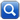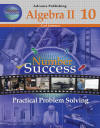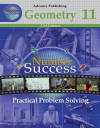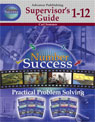﻿ Practical Problem-Solving Mathematics DVD Program, Teachers: Winners Presidential Award for Excellence in Mathematics National Science FoundationNumber Success
A Motivational Practical Math Program from Addition to Trigonometry
Coming Soon!!
Over 50 Hours of Video!

Use Number Success Now

View a One Minute Video Introduction to the Series
Program Description              Number Success Endorsements

 Addition  Book 1 Adding various place numbers The number system Saying, writing and adding large numbers Solving measurements Conversion problems Adding money and problem solving Subtraction  Book 2 Subtracting various place numbers Subtraction with borrowing Understanding numbers Problem solving with addition and subtraction Check writing and bank deposits Comparing prices Multiplication  Book 3 Multiplication table from 1 to 12 Multiplying with various place numbers Carrying over numbers Multiplication word problems Addition, subtraction and multiplication problems Multiplication and money Division  Book 4 Dividing from 1 to 12 Comparing multiplication with division Dividing with various place numbers Division problem solving Multiplication and division problem solving Estimating numbers Addition, subtraction, multiplication and division problems Fractions  Book 5 Understanding and comparing fractions Writing equivalent fractions Raising and reducing fractions Adding, subtracting, multiplying and dividing various fraction problems Rulers and measurements Money problems Decimals and Percents  Book 6 Comparing fractions, decimals and percents Adding, subtraction, multiplying and dividing decimals Changing fractions, decimals and percents Fraction, tenth, hundredth and metric rulers Problem solving with fractions, decimals and percents Calculator Achievement  Book 7 Calculator operation instructions Adding, subtracting, multiplying and dividing with a calculator Using estimating skills Weights, measures and interest problems Using memory keys Converting English and metric weights and measurements Solving fraction, decimal and percentage problems with a calculator Life Skills Math Power  Book 8 Area and perimeter of rectangles, squares, triangles and circles Volume of rectangular solids, cubes, and cylinders Converting measurements Finding averages and graph problem solving Figuring wages and commissions Using a tax-rate schedule Owning your own business: expenses, earnings and profits Purchasing, invoicing and packing lists Simple, compound and daily compound interest rates Credit and monthly installment payments Operating a checking account and paying telephone and electric service bills Buying and owning a house: closing costs, points and operating costs Car rentals, mileage tables and auto depreciation Algebra 1  Book 9 Signed numbers and the number line Adding, subtracting, multiplying, and dividing signed numbers and equations Practical applications for signed numbers, powers, and square roots Problem solving using formulas Application of algebraic expressions using formulas Solving exponent equationsSearch Inside Book Algebra 2  Book 10 Solving equations with multiple unknowns Adding, subtracting, multiplying, and dividing algebraic terms Ratio, proportion, and probability Equations with symbols of inclusion Solving quadratic equations by factoring Word problems as mathematical phrases and equations Distance, rate, and time problems Consecutive integer problemsSearch Inside Book Geometry  Book 11 Formulas and geometric symbols Rectangular coordinates Graphing linear equations and calculating the slope of a line Lines and angles Angle problem solving Perimeter and areas of triangles and quadrilaterals Pythagorean theorem Circumference and area of circles Solid geometry Practical geometric problem solvingSearch Inside Book Trigonometry  Book 12 Formulas and solutions for finding angles and sides of right and oblique-angled triangles Ratio and angles and sides of triangles Using a calculator for finding angles and sides of right and oblique-angled triangles Converting minutes and seconds Locating X and Y positions Practical problem solving for right and oblique-angled trianglesSupervisor's Guide and Placement Exams Instructions for supervisors Instructions for students Placement evaluation for books 1 to 7 Placement evaluation for books 1 to 11 Certificate of achievement